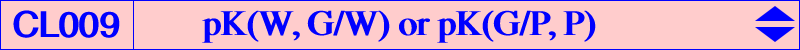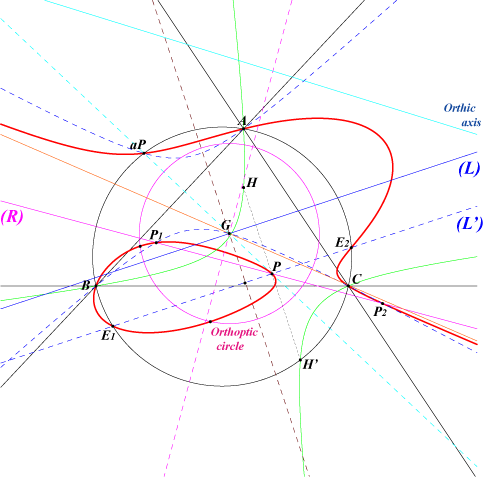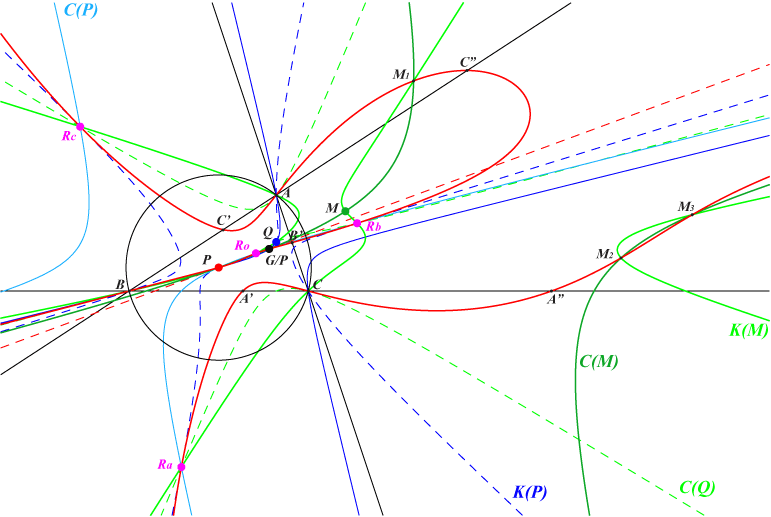Given a pole W = (p : q : r) of isoconjugation, pK(W, G/W) is the locus of point M such that there is a pK with same pole W having its asymptotes parallel to the cevian lines of M. These are the only pKs such thar the polar conics of the pivot and isopivot are homothetic. See below. The pivot P = G/W is the cevian quotient of G and W or the G-Ceva conjugate of W : it is the center of the circum-conic with perspector W. When W is G or when W lies on the Steiner in-ellipse, it decomposes into three lines. pK(W,G/W) is always a pK+ and its asymptotes concur at G. Its equation is : ∑ p(– p + q + r) x (r y^2 – q z^2) = 0. pK(G/P, P) contains P and its anticomplement aP (the isopivot). The tangents at the common points of the cubic and the line Lp = P-G/P concur at G hence the polar conic of G is the union of the line at infinity and the line Lp. Lp contains P and two other points P1, P2 on the cubic and on the circum-conic passing through G and aP. The most interesting example is obtained when W = K = X(6) since pK(W,G/W) is the McCay cubic. In other words, the McCay cubic is the locus of point M such that there is an isogonal pK with asymptotes parallel to the cevian lines of M. For example, when M = H = X(4), the isogonal pK is the Darboux cubic, when M = O = X(3), the isogonal pK is K158. The table below gives a selection of pK(W, G/W) according to the pole W.pole W pivot W/G cubic remark / X(i) on the cubic X(1) X(9) X(7), X(9), X(366) X(2) X(2) union of the medians X(3) X(6) X(6), X(69) X(4) X(1249) K159 X(253) X(6) X(3) K003 McCay cubic the only equilateral pK(W,G/W) X(9) X(1) K157 X(8), X(188), X(979) X(32) X(206) K160 X(6), X(66) X(523) X(115) K392 see below X(1249) X(4) K329 X(20) X(1637) X(3258) K601 X(476)Cubics with pole on the orthic axis We may consider the polar conic of G as a degenerate circle and, in general, there are no other points in the plane having a circular polar conic. But when W lies on the orthic axis or, equivalently, P lies on the nine point circle, there is a line (L) of points – we call the circular line of the cubic – having a circular polar conic as in Table 47. Note that, in this case, P is the center of the rectangular circum-hyperbola (H) which is the W-isoconjugate of the line at infinity. W is its perspector and the reflection H' of aP in O (or H in P) is its last point on the circum-circle. (L) contains G and the W-isoconjugate of H'. It is the W-isoconjugate of the circum-conic through H' and W. The cubic meets the circumcircle at A, B, C, aP and two other points E1, E2 on the parallel (L') at P to the line (L). (L') is the W-isoconjugate of the circum-conic through H' and aP. When W traverses the orthic axis, (L') envelopes the McBeath conic, the in-conic with foci O and H. All these circular polar conics form a pencil of circles whose radical axis is the line (R) passing through P and W. They are obviously centered on the perpendicular (C) at G to (R). Note that (R) is the W-isoconjugate of the circum-conic through G and aP. Hence, this conic meets (R) at two isoconjugate points P1, P2 on the cubic. The tangents at P, P1, P2 to the cubic concur at G and the polar conic of G is the union of (R) and the line at infinity (remind that the asymptotes of the cubic also concur at G). It follows that there are three points (one at least is real) on the cubic having a circular polar conic. The polar conic of the W-isoconjugate of H' (the infinite point of (L)) is the orthoptic circle (S) of the Steiner in-ellipse. Thus, any circular polar conic is centered on (C) and contains the common points of (S) and (R). Furthermore, (S) meets the cubic at six (not necessarily real) points having tangents parallel to (L).K392 = pK(X523, X115) is probably the most interesting example of such cubic. See also K601 = pK(X1637, X3258). See CL049 for other pKs with circular polar conics.Polar conics of pivot and isopivot of a pK Let Q = P* be the isopivot of pK(W, P). Denote by C(P) and C(Q) the polar conics of P and Q in pK(W, P). Recall that : • C(P) is the diagonal conic passing through P, its three harmonic associates (the vertices of the anticevian triangle of P) which is tangent at P to the line PQ. C(P) also contains the (not always real) four fixed points of the isoconjugation (actually the square roots of W). • C(Q) is the circum-conic passing through P, Q. It is tangent at Q to the line through Q and P/Q. C(P) and C(Q) have four common points which are the poles of the line PQ in the cubic pK(W, P). After some calculations, we obtain the following results : • C(P) and C(Q) have at least one common point on the line at infinity if and only if W lies on the cubic psK(P x G/P, G, G/P) which is a nodal circum-cubic with node G/P, actually the Deléham cubic Del(G/P) of CL033. With P = (u : v : w), the equation of psK(P x G/P, G, G/P) is : 2(u – v)(v – w)(w – u) x y z + ∑ u^2 (– u + v + w)(y – z) y z = 0. • C(P) and C(Q) have two common points on the line at infinity if and only if W = G/P giving precisely the cubics pK(G/P, P). In other words, these polar conics are homothetic and meet at P and G/P. • These polar conics are homothetic rectangular hyperbolas when P lies on the Thomson cubic K002 in which case G/P also lies on K002. For example, if P = O = X(3) then G/P = K = X(6), Q = H = X(4) and the cubic pK(G/P, P) becomes the McCay cubic K003. The conics C(P) and C(Q) are the Stammler and Jerabek hyperbolas meeting at O, K and X(2574), X(2575) at infinity.Related quartics similar to Q002 Let P be a point different of G. Let Ω = G/P and Q = aP (anticomplement of P) be the pole and the isopivot of the cubic K(P) = pK(Ω, P). The polar conics C(P) and C(Q) of P and Q have the same points J1, J2 at infinity and meet again at P and Ω. They define a pencil of conics and let C(M) be the conic of the pencil that passes through a point M. Now, if we take M on the line PQ, we can associate to any conic C(M) the pivotal cubic K(M) with pole Ω, pivot M which obviously must contain P, Q and M. When M varies, K(M) and C(M) meet at 6 points namely the fixed points P, Ω, four other variable points M and M1, M2, M3. The locus of these three latter points is a quartic Q(P) that contains : A, B, C which are inflexion points with tangents passing through P, the infinite points of C(P) (or C(Q)) and those of the circum-conic C(Ω) with perspector Ω, P, Ω = G/P, two other (real or not) points on the line PΩ analogous to the isodynamic points (obtained when P = O). These points lie on a circum-conic passing through G and with center on the Steiner in-ellipse. the (real or not) square roots Ro, Ra, Rb, Rc of Ω which also lie on C(P), K(P) and where the tangents pass through P, the 6 traces (other than A, B, C) of the sidelines of the complete quadrilateral RoRaRbRc on the sidelines of ABC,With P = u : v : w, the equation of Q(P) is : ∑ x^3(w y - v z) / [u(-u+v+w)] = 0 or equivalently ∑ u^2 (-u+v+w) [w (-w+u+v) y^2 - v (-v+w+u) z^2]y z. The most remarkable quartic is Q002 obtained when P = O.